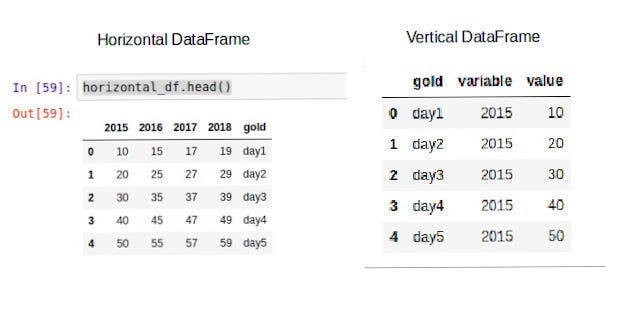# Data Science Toolkit (Concepts + Code)

Hi folks !! In this post, i will discuss about basic tools and software that one can use to solve a data science problem . If you are new to ML or Data Science or Statistics, Feel free to check out my other blog on ML by clicking on the link below.

Machine Learning 101 [Part1] (concepts + Examples)

### What is a Data Science Toolkit ?

Well, Data science toolkit is nothing but a list of functions / modules / packages / frameworks /software that can really help a data scientist to solve a problem. Sometimes you have these functions / packages available in form of 3rd party packages or software and sometimes you are required to create your own. That’s why a True Data Scientist is a mix of ( Statistician and a Programmer ).

NOTE : I am already assuming that you are well verse with Statistics and you have a fair knowledge of Python .[ If not, Then go and learn Stats and programming first 🙂 ] So, Without wasting time lets get started .

### Jupyter Notebook

The Jupyter Notebook is an open-source web application that allows you to create and share documents that contain live code, equations, visualizations and narrative text. It is widely used in the data science community. You can download jupyter notebook from the link : https://jupyter.org/install .

Lets look at some of the shortcut command’s of this notebook .

1. ctrl + Enter : Run the Selected Cells
2. shift + Enter: Run the current cell and select below
3. Alt + Enter : Run the current cell and Insert a new cell below .
4. M : To change the cell type to Markdown
5. Y : To change the cell type to Code
6. A : Insert a cell Above
7. B : Insert a cell below

### Numpy

NumPy is the fundamental package for scientific computing with Python. It is very powerful and is widely used in solving data science problems . Lets look at how to use this library with the help of a coding example.

The above code is pretty much self-explanatory, I am simply creating a numpy array of 1-dimension and 2-dimensions by passing a list of values in it , checking its data type using dtype method and checking the dimensions of the numpy array using shape method. Then, i am reshaping it using reshape method by passing in the rows and column values i want my array to reshape in. Slicing in numpy array is easily done by using the below syntax: numpy_array[row_to_extract , column_to_extract] or numpy_array[start_row_index:end_row_index,start_col_index:end_col_index]

### Pandas

Pandas is an open- source library providing high-performance, easy-to-use data structures and data analysis tools for the Python. To be honest, It is just like excel or sql but a little advanced and a little better. Lets look at some code examples . you can get the data by clicking on the link below .

Lets look at the three functions i have used in the above code .

1. read_csv : This is used to convert a csv file into a dataframe.
2. head : This is used to find the top 5 rows in the dataset/dataframe .
3. shape : Shape method will return the number of rows and columns of a dataframe.

#### Subsetting:

Q1. Extract only those rows where column_name: ‘mpg’ is greater than 30 .

Q2. Extract only those rows where column_name: ‘origin’ is equal to ‘Asia’

Q3. Select only top 20 rows of the data/dataframe

Lets look at the syntax of above code .

1. loc[] : loc means location and loc method is used to access a group of rows and columns by labels.
2. iloc[]: iloc means index location and iloc method is used to access a group of rows and columns by their indexes.

#### Reshaping DataFrameOutput : Implementation of melt() method . DataFrame Converted to vertical format from horizontal format

Lets look at the functions used in the above code .

1. DataFrame : It is used to convert a dictionary to a dataframe.
2. melt: This method unpivots a dataframe from wide format to long format, optionally leaving identifier variables set.

### Plotly

Plotly is a plotting library and is used to plot graphs. It really helps in data visualisation and makes a data scientist job so easy. With plotly, a Data Scientist can visualise the given data in a very very easy way. I recently wrote a post “Data Visualization with plotly (Code)”. Feel free to check it out by clicking on the link below .

Data Visualization using Plotly (Code)

### Scikit-Learn / Sklearn

Scikit-learn is a free software machine learning library for the Python. It provides a lot of machine learning algorithms with few lines of code. According to me, This library is a blessing to all data scientist. Lets look at a coding example .

I hope you liked my post ! If yes, Please, give it a clap. It would encourage me to write more and if you are new to data science, feel free to check out my post on “Descriptive Stats (Concepts + Code )” by clicking on the link below .

Descriptive Stats (Concepts + Code)

Thanks for reading my post. And don’t forget to Clap, Share and Follow .Data Science Toolkit (Concepts + Code) was originally published in HackerNoon.com on Medium, where people are continuing the conversation by highlighting and responding to this story.